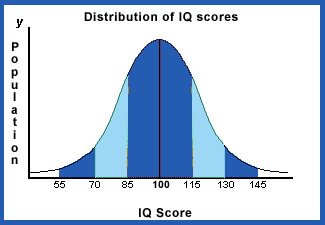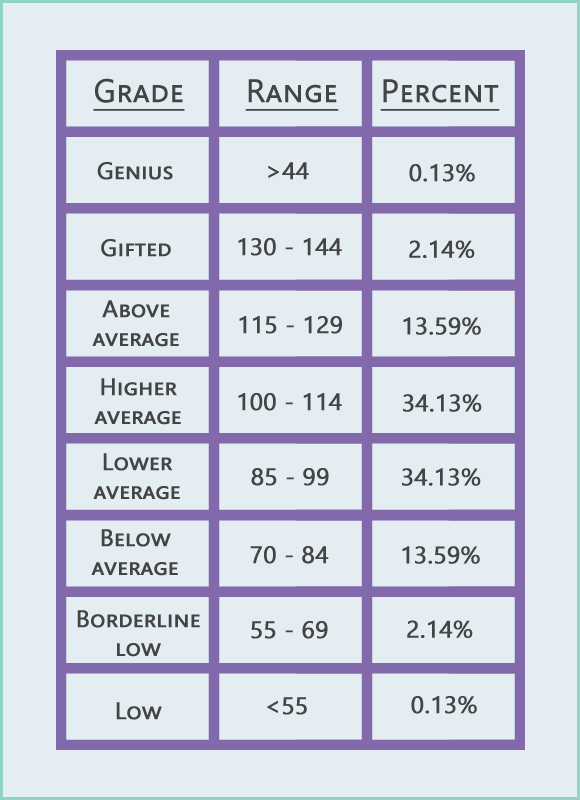# IQ Test Labs### Sample personalized IQ report for Jane

Thank you for taking the test.

The average score for all test takers is 100.

You scored higher than 64 % of all the people that took this test.

General statistics:

Total number of questions: 30The IQ Test Labs scale is equivalent to the Wechsler scale

(mean=100 and standard deviation=15)

The bell curve (also called a "normal curve" or "normal distribution") is a graph that shows approximately how much of the population falls into each IQ range. In theory, if we tested everyone in the world with a traditional IQ test, most people would score in the " Average" range. A smaller number would score moderately below average and moderately above average. Very high and very low scores are rare.

The scores and percentiles above apply to tests that have a standard deviation of 15 points. The Stanford Binet Fourth Edition (Binet FE) has a standard deviation of 16 and the Wechsler scales (such as the WPPSI-R, the WISC-III, and the WAIS-III) have a standard deviation of 15. Therefore, for different scales the percentages may vary.